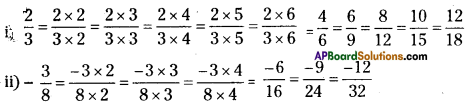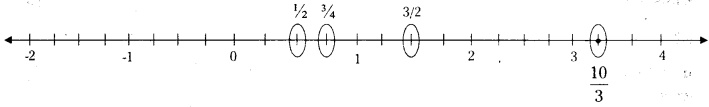# HSSlive: Plus One & Plus Two Notes & Solutions for Kerala State Board

## AP Board Class 7 Maths Chapter 2 Fractions Decimals and Rational Numbers Ex 7 Textbook Solutions PDF: Download Andhra Pradesh Board STD 7th Maths Chapter 2 Fractions Decimals and Rational Numbers Ex 7 Book AnswersAP Board Class 7 Maths Chapter 2 Fractions Decimals and Rational Numbers Ex 7 Textbook Solutions PDF: Download Andhra Pradesh Board STD 7th Maths Chapter 2 Fractions Decimals and Rational Numbers Ex 7 Book Answers

## Andhra Pradesh State Board Class 7th Maths Chapter 2 Fractions Decimals and Rational Numbers Ex 7 Books Solutions

 Board AP Board Materials Textbook Solutions/Guide Format DOC/PDF Class 7th Subject Maths Chapters Maths Chapter 2 Fractions Decimals and Rational Numbers Ex 7 Provider Hsslive

## How to download Andhra Pradesh Board Class 7th Maths Chapter 2 Fractions Decimals and Rational Numbers Ex 7 Textbook Solutions Answers PDF Online?

2. Click on the Andhra Pradesh Board Class 7th Maths Chapter 2 Fractions Decimals and Rational Numbers Ex 7 Answers.
3. Look for your Andhra Pradesh Board STD 7th Maths Chapter 2 Fractions Decimals and Rational Numbers Ex 7 Textbooks PDF.
4. Now download or read the Andhra Pradesh Board Class 7th Maths Chapter 2 Fractions Decimals and Rational Numbers Ex 7 Textbook Solutions for PDF Free.

## AP Board Class 7th Maths Chapter 2 Fractions Decimals and Rational Numbers Ex 7 Textbooks Solutions with Answer PDF Download

Find below the list of all AP Board Class 7th Maths Chapter 2 Fractions Decimals and Rational Numbers Ex 7 Textbook Solutions for PDF’s for you to download and prepare for the upcoming exams:

Question 1.
Write any three equivalent rational numbers to each of the following
i) 23
ii) −38
Solution:Question 2.
What is the equivalent rational number for −1536 with
(i) denominator 12
(ii) numerator 75?
Solution:
i) −1536=−15÷336÷3=−512
ii) −1536=−15×536×5=−75180

Question 3.
Mark the following rational numbers on the number line.
(i) 12
(ii) 34
(iii) 32
(iv) 103
Solution:## Andhra Pradesh Board Class 7th Maths Chapter 2 Fractions Decimals and Rational Numbers Ex 7 Textbooks for Exam Preparations

Andhra Pradesh Board Class 7th Maths Chapter 2 Fractions Decimals and Rational Numbers Ex 7 Textbook Solutions can be of great help in your Andhra Pradesh Board Class 7th Maths Chapter 2 Fractions Decimals and Rational Numbers Ex 7 exam preparation. The AP Board STD 7th Maths Chapter 2 Fractions Decimals and Rational Numbers Ex 7 Textbooks study material, used with the English medium textbooks, can help you complete the entire Class 7th Maths Chapter 2 Fractions Decimals and Rational Numbers Ex 7 Books State Board syllabus with maximum efficiency.

## FAQs Regarding Andhra Pradesh Board Class 7th Maths Chapter 2 Fractions Decimals and Rational Numbers Ex 7 Textbook Solutions

#### Can we get a Andhra Pradesh State Board Book PDF for all Classes?

Yes you can get Andhra Pradesh Board Text Book PDF for all classes using the links provided in the above article.

## Important Terms

Andhra Pradesh Board Class 7th Maths Chapter 2 Fractions Decimals and Rational Numbers Ex 7, AP Board Class 7th Maths Chapter 2 Fractions Decimals and Rational Numbers Ex 7 Textbooks, Andhra Pradesh State Board Class 7th Maths Chapter 2 Fractions Decimals and Rational Numbers Ex 7, Andhra Pradesh State Board Class 7th Maths Chapter 2 Fractions Decimals and Rational Numbers Ex 7 Textbook solutions, AP Board Class 7th Maths Chapter 2 Fractions Decimals and Rational Numbers Ex 7 Textbooks Solutions, Andhra Pradesh Board STD 7th Maths Chapter 2 Fractions Decimals and Rational Numbers Ex 7, AP Board STD 7th Maths Chapter 2 Fractions Decimals and Rational Numbers Ex 7 Textbooks, Andhra Pradesh State Board STD 7th Maths Chapter 2 Fractions Decimals and Rational Numbers Ex 7, Andhra Pradesh State Board STD 7th Maths Chapter 2 Fractions Decimals and Rational Numbers Ex 7 Textbook solutions, AP Board STD 7th Maths Chapter 2 Fractions Decimals and Rational Numbers Ex 7 Textbooks Solutions,
Share: